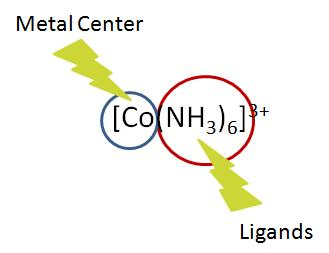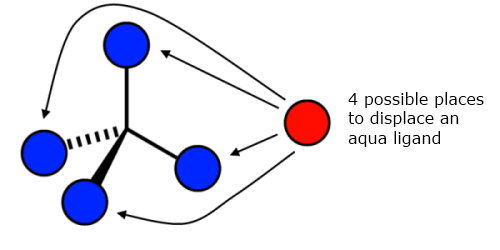# Complex-Ion Equilibria

$$\newcommand{\vecs}{\overset { \rightharpoonup} {\mathbf{#1}} }$$ $$\newcommand{\vecd}{\overset{-\!-\!\rightharpoonup}{\vphantom{a}\smash {#1}}}$$$$\newcommand{\id}{\mathrm{id}}$$ $$\newcommand{\Span}{\mathrm{span}}$$ $$\newcommand{\kernel}{\mathrm{null}\,}$$ $$\newcommand{\range}{\mathrm{range}\,}$$ $$\newcommand{\RealPart}{\mathrm{Re}}$$ $$\newcommand{\ImaginaryPart}{\mathrm{Im}}$$ $$\newcommand{\Argument}{\mathrm{Arg}}$$ $$\newcommand{\norm}{\| #1 \|}$$ $$\newcommand{\inner}{\langle #1, #2 \rangle}$$ $$\newcommand{\Span}{\mathrm{span}}$$ $$\newcommand{\id}{\mathrm{id}}$$ $$\newcommand{\Span}{\mathrm{span}}$$ $$\newcommand{\kernel}{\mathrm{null}\,}$$ $$\newcommand{\range}{\mathrm{range}\,}$$ $$\newcommand{\RealPart}{\mathrm{Re}}$$ $$\newcommand{\ImaginaryPart}{\mathrm{Im}}$$ $$\newcommand{\Argument}{\mathrm{Arg}}$$ $$\newcommand{\norm}{\| #1 \|}$$ $$\newcommand{\inner}{\langle #1, #2 \rangle}$$ $$\newcommand{\Span}{\mathrm{span}}$$

When a metal ion reacts with a Lewis base in solution a complex ion is formed. This reaction can be described in terms of chemical equilibria.

## Introduction

A complex ion is comprised of two important parts: the central atom and its surrounding ligands. The central atom can be any metallic ion (usually a transition metal). The ligands are any combination of anions that can donate an electron pair, effectively meaning they are all Lewis bases. When combined they form coordinate covalent bonds.## Formation Constant

In general, chemical equilibrium is reached when the forward reaction rate is equal to the reverse reaction rate and can be described using an equilibrium constant, $$K$$. Complex ion equilibria are no exception to this and have their own unique equilibrium constant. This formation constant, $$K_f$$, describes the formation of a complex ion from its central ion and attached ligands. This constant may be called a stability constant or association constant; the units depend on the specific reaction it is describing. Common metal/ligand $$K_f$$ values are tabulated in Table E4.

At its most basic level, $$K_f$$ can be explained as the following, where $$M$$ is a metal ion, $$L$$ is a ligand, and $$x$$ and $$y$$ are coefficients:

$K_f = \dfrac{[M_xL_y]} {[M]^x[L]^y}$

$$K_f$$ values are very large in magnitude for complex ion formation reactions heavily favor the product and very small for poorly forming complex ions. For example, observe the formation reaction of the dicyanoargentate(I) complex ion

$\ce{Ag^{+}(aq) + 2CN^{-} (aq) <=> Ag(CN)2^{-}(aq)} \nonumber$

and the resulting formation constant expression:

$K_f = \dfrac{[Ag(CN)_2^-]}{[Ag^{+}][CN^{-}]^{2}} = 5.6 \times 10^{18}$

The larger the $$K_f$$ value of a complex ion, the more stable it is. Due to how large formation constants often are it is not uncommon to see them listed as logarithms in the form $$\log K_f$$. You may also see them in the form of a dissociation constant, $$K_d$$ (which should not be confused with pKa). This is merely the inverse value of the formation constant and as such describes the instability of a complex ion. It may help to think of $$K_f$$ and $$K_d$$ as stability and instability constants, respectively.

Using the above example, the dissociation constant expression would be:

$$K_f = \dfrac{1}{K_d}$$

$$K_{d} = \dfrac{1}{K_f} = \dfrac{[Ag^{+}][CN^{-}]^{2}}{[Ag(CN)_2]^{-}} = 1.8 \times 10^{-19}$$

In logarithm form $$K_f$$and $$K_d$$ would be:

$$\log K_{f} = \log(5.6 \times 10^{18}) = 18.7$$

$$\log K_{d} = \log(1.8 \times 10^{-19}) = -18.7$$

Example $$\PageIndex{1}$$: Stepwise Equilbria

One might assume that the formation of complex ions is a one step process, however this is not always the case. As an example let's look at the formation of tetraamminecopper(II) ion in solution:

$\ce{[Cu(H2O)4]^{2+}(aq) + 4NH3 (aq) <=> [Cu(NH3)4]^{2+}(aq) + 4H2O(l)} \nonumber$

The reason for using the hydrated form of the copper ion is that cations in solution usually exist in their hydrated form. It is because of this that the creation of tetraamminecopper(II) ion is a multi-step process, the ammine ligands have to displace the existing aqua ligands. In calculations however, the concentration of water and the number of aqua ligands are effectively constant meaning that they can be safely ignored. Each step of this process has its own formation constant, and as with other chemical equilibria, the overall reaction constant is the product of these stepwise formation constants.

Step 1:

$[Cu(H_2O)_4]^{2+}_{(aq)} + NH_{3 \; (aq)} \rightleftharpoons [Cu(H_2O)_3(NH_3)]^{2+}_{(aq)} + H_2O_{(l)} \tag{step 1}$

$K_{1} = \dfrac{[[Cu(H_2O)_3(NH_3)]^{2+}]}{[[Cu(H_2O)_4]^{2+}][NH_3]} = 1.9 \times 10^4 \nonumber$

Step 2:

$[Cu(H_2O)_3(NH_3)]^{2+}_{(aq)} + NH_{3 \; (aq)} \rightleftharpoons [Cu(H_2O)_2(NH_3)_2]^{2+}_{(aq)} + H_2O_{(l)} \tag{step 2}$

$K_{2} = \dfrac{[[Cu(H_2O)_2(NH_3)_2]^{2+}]}{[[Cu(H_2O)_3(NH_3)]^{2+}][NH_3]} = 3.9 \times 10^3 \nonumber$

Step 3:

$[Cu(H_2O)_2(NH_3)_2]^{2+}_{(aq)} + NH_{3 \; (aq)} \rightleftharpoons [Cu(H_2O)(NH_3)_3]^{2+}_{(aq)} + H_2O_{(l)} \tag{step 3}$

$K_{3} = \dfrac{[[Cu(H_2O)(NH_3)_3]^{2+}]}{[[Cu(H_2O)_2(NH_3)_2]^{2+}][NH_3]} = 1.0 \times 10^3 \nonumber$

Step 4:

$[Cu(H_2O)(NH_3)_3]^{2+}_{(aq)} + NH_{3 \; (aq)} \rightleftharpoons [Cu(NH_3)_4]^{2+}_{(aq)} + H_2O_{(l)} \tag{step 4}$

$K_{4} = \dfrac{[[Cu(NH_3)_4]^{2+}]}{[[Cu(H_2O)(NH_3)_3]^{2+}][NH_3]} = 1.5 \times 10^2 \nonumber$

Total

\begin{align*} K_f &= K_{1} \times K_{2} \times K_{3} \times K_{4} \\[4pt] &= \dfrac{[[Cu(H_2O)_3(NH_3)]^{2+}]}{[[Cu(H_2O)_4]^{2+}][NH_3]} \times \dfrac{[[Cu(H_2O)_2(NH_3)_2]^{2+}]}{[[Cu(H_2O)_3(NH_3)]^{2+}][NH_3]} \times \dfrac{[[Cu(H_2O)(NH_3)_3]^{2+}]}{[[Cu(H_2O)_2(NH_3)_2]^{2+}][NH_3]} \times \dfrac{[[Cu(NH_3)_4]^{2+}]}{[[Cu(H_2O)(NH_3)_3]^{2+}][NH_3]} \\[4pt] &= \dfrac{[[Cu(NH_3)_4]^{2+}]}{[[Cu(H_2O)_4]^{2+}][NH_3]^{4}} \\[4pt] &= 1.1 \times 10^{13} \end{align*}

If you wanted to find the formation constant of one of the intermediate steps, you would simply take the product of the $$K$$ values up until that point. For example the formation constant of $$[Cu(H_2O)_2(NH_3)_2]^{2+}$$ would be $$\beta_2 = K_{1} \times K_{2} = 7.4 \times 10^7$$. As seen here and above the standard notation for these values is a lowercase beta.

Example $$\PageIndex{2}$$: Using Formation Constants as a Metric of Stability

Based on the given values, rank the following complex ions from most to least stable.

• $$[Cr(OH)_4]^{-}$$ $$K_f = 8.0 \times 10^{29}$$
• $$[Ni(CN)_4]^{2-}$$ $$\log(K_d) = -31.3$$
• $$[Co(NH_3)_6]^{3+}$$ $$K_d = 2.2 \times 10^{-34}$$
• $$[Cu(CN)_4]^{3-}$$ $$\log(K_f) = 30.3$$

Solution

The key to solving this problem is to convert all of the given values into the same form. In this case we will convert all of them into the form $$K_f$$ though any other form could be used as long as you are consistent.

• $$[Cr(OH)_4]^{-}$$ $$K_f = 8.0 \times 10^{29}$$
• $$[Ni(CN)_4]^{2-}$$ $$\log(K_d) = -31.3 \longrightarrow K_f = 2.0 \times 10^{31}$$
• $$[Co(NH_3)_6]^{3+}$$ $$K_d = 2.2 \times 10^{-34} \longrightarrow K_f = 4.5 \times 10^{33}$$
• $$[Cu(CN)_4]^{3-}$$ $$\log(K_f) = 30.3 \longrightarrow K_f = 2.0 \times 10^{30}$$

After converting it is easy to rate the ions in terms of stability. From most to least stable:

$[Co(NH_3)_6]^{3+} > [Ni(CN)_4]^{2-} > [Cu(CN)_4]^{3-} > [Cr(OH)_4]^{-}. \nonumber$

You may notice that each stepwise formation constant is smaller than the one before it. This decreasing trend is due to the effects of entropy, causing each step to be progressively less likely to occur. You can think of this in the following way, continuing with the previous example:When the first ammine ligand goes to displace an aqua ligand it has four sites from which to choose from, making it "easier" to displace one. Yet with every step the number of sites decreases making it increasingly more difficult. As always though, there are exceptions to this rule. If the values do not continually decrease then the structure of the complex ion likely changed during one of the steps.

## Chelation Effect

Generally, complex ions with polydentate ligands have much higher formation constants than those with monodentate ligands. This additional stability is known as the chelation effect. For example, the formation of the bis(ethylenediamine)copper(II) ion is identical to the tetraamminecopper(II) ion above except the ethylenediamine ligands displace two aqua ligands at a time. Observe the differences in formation constant (Table E4):

Complex Ion Kf
$$[Cu(NH_3)_4]^{2+}$$ $$1.1 \times 10^{13}$$
$$[Cu(en)_2]^{2+}$$ $$1.0 \times 10^{20}$$

Example $$\PageIndex{3}$$

Of $$\ce{[Ni(en)3]^{2+}}$$, $$\ce{[Ni(EDTA)]^{2-}}$$, and $$\ce{[Ni(NH3)6]^{2+}}$$ which would you expect to have the largest $$K_f$$ value? The smallest? Explain.

Solution

This is a question involving the effects of chelation. When the complex ion with EDTA is formed, the EDTA ligand displaces six aqua ligands at once. Ethylenediamine ligands displace two at a time and ammine ligands displace one at a time. Based on this we would expect $$\ce{[Ni(EDTA)]^{2-}}$$ to be the most stable and $$\ce{[Ni(NH3)6]^{2+}}$$ to be the least stable.

## Solubility Effects

The formation of complex ions can sometimes explain the solubility of certain compounds in solution. For example, let's say we have a saturated solution of silver bromide ($$AgBr$$) which also contains additional undissolved solid silver bromide. If high enough concentrations of ammonia are added to the solution, the solid silver bromide will dissolve despite the solution previously having been saturated. This is because of the formation diammineargentate(I) ion, illustrated by the following chemical equation:

$\ce{AgBr(s) + 2NH3 (aq) \rightarrow [Ag(NH3)2]^{+}(aq) + Br^{-}(l)} \nonumber$

## References

1. Beck, Mihály T. Chemistry of Complex Equilibria. Revised ed. New York: Halsted Press, 1990.
2. Brown, Theodore, Eugene Lemay, Bruce Bersten, and Catherine Murphey. Chemistry: The Central Science. 10th ed. Andalusia, Alabama: Pearson Publications Company, 2006.
3. Petrucci, Harwood, Herring, and Madura. General Chemistry: Principles and Modern Applications. 9th ed. Upper Saddle River, New Jersey: Pearson Education, 2007.
4. Wilkinson, Geoffrey, Robert D. Gillard, and Jon A. McCleverty, eds. Comprehensive Coordination Chemistry: The Synthesis, Reactions, Properties & Applications of Coordination Compounds. First ed. Vol. 1. Oxford, England: Pergamon Press, 1987. N. pag. 7 vols.

Example $$\PageIndex{4}$$

The creation of $$\ce{[Ni(en)_3]^{2+}}$$ is a three step process with the following stepwise formation constants $$K_1 = 3.3 \times 10^{7}$$, $$K_2 = 1.9 \times 10^{6}$$, and $$K_3 = 1.8 \times 10^{4}$$. Using this information find the overall formation constant and the formation constant for each of the intermediate steps.

Solution

Take the product of the stepwise formation constants up until the step you are trying to find.

$$[Ni(H_2O)_4(en)]^{2+}$$ $$\beta_1 = K_1 = 3.3 \times 10^{7}$$

$$[Ni(H_2O)_2(en)_2]^{2+}$$ $$\beta_2 = K_1 \times K_2 = 6.8 \times 10^{13}$$

$$[Ni(en)_3]^{2+}$$ $$\beta_3 = K_f = K_1 \times K_2 \times K_3 = 1.1 \times 10^{18}$$